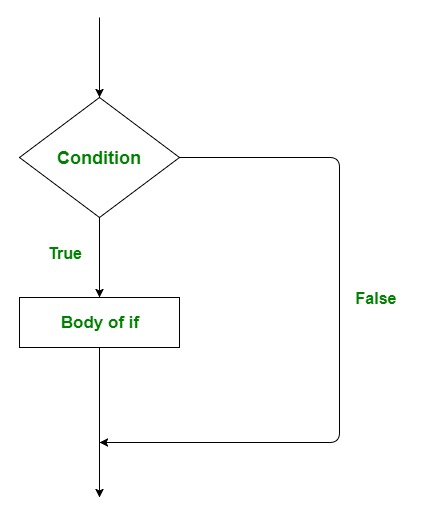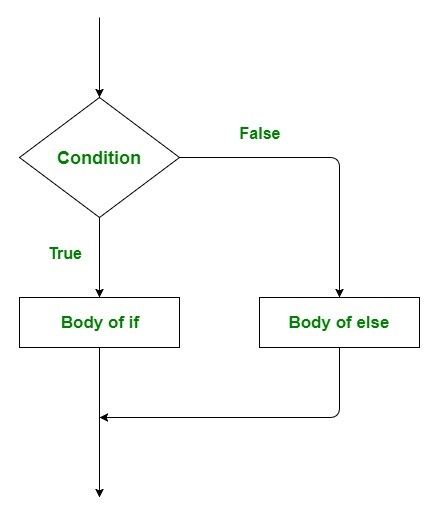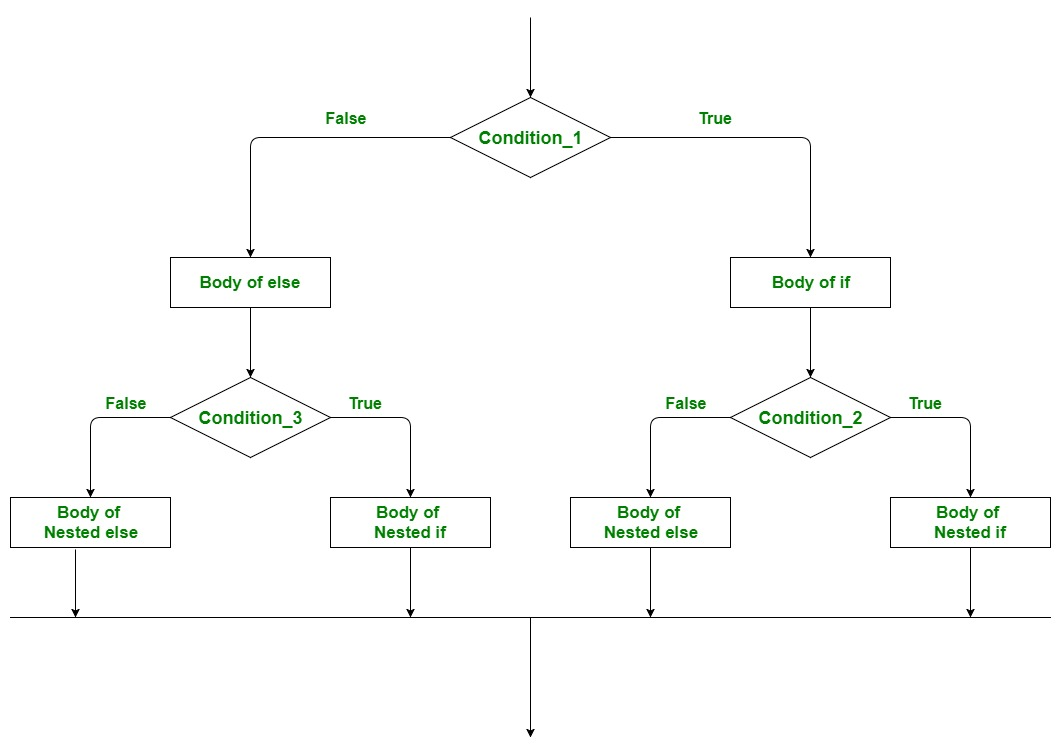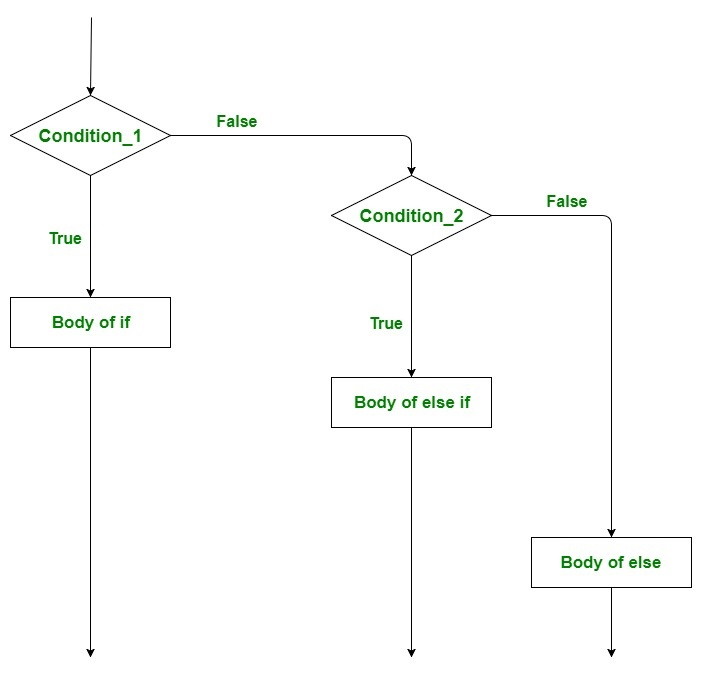# Scala | Decision Making (if, if-else, Nested if-else, if-else if)

Decision making in programming is similar to decision making in real life. In decision making, a piece of code is executed when the given condition is fulfilled. Sometimes these are also termed as the Control flow statements. Scala uses control statements to control the flow of execution of the program based on certain conditions. These are used to cause the flow of execution to advance and branch based on changes to the state of a program.

The conditional statements of Scala are:

#### if statement

if” statement is the simplest decision making statements among all decision making statements. In this statement, the block of code is executed only when the given condition is true and if the condition is false then that block of code will not execute.

Syntax:

```if(condition)
{
// Code to be executed
}
```

Here, condition after evaluation will be either true or false. if statement accepts boolean values – if the value is true then it will execute the block of statements under it.
If we do not provide the curly braces ‘{‘ and ‘}’ after if(condition) then by default if statement will consider the immediate one statement to be inside its block.
Example:

```if(condition)
statement1;
statement2;

// Here if the condition is true, if block
// will consider only statement1 to be inside
// its block.
```

Flow Chart:Example:

 `// Scala program to illustrate the if statement ` `object` `Test { ` `     `  `// Main Method ` `def` `main(args``:` `Array[String]) { ` `     `  `    ``// taking a variable ` `    ``var` `a``:` `Int ``=` `50` ` `  `    ``if` `(a ``>` `30``)  ` `    ``{ ` ` `  `        ``// This statement will execute as a > 30 ` `        ``println(``"GeeksforGeeks"``) ` `    ``} ` `} ` `} `

Output:

`GeeksforGeeks`

#### if-else statement

The if statement alone tells us that if a condition is true it will execute a block of statements and if the condition is false it won’t. But what if we want to do something else if the condition is false. Here comes the else statement. We can use the else statement with if statement to execute a block of code when the condition is false.

Syntax:

```if (condition)
{
// Executes this block if
// condition is true
}

else
{
// Executes this block if
// condition is false
}
```

Flow Chart:Example:

 `// Scala program to illustrate the if-else statement ` `object` `Test { ` `     `  `// Main Method ` `def` `main(args``:` `Array[String]) { ` `     `  `    ``// taking a variable ` `    ``var` `a``:` `Int ``=` `650` ` `  `    ``if` `(a ``>` `698``)  ` `    ``{ ` ` `  `        ``// This statement will not  ` `        ``// execute as a > 698 is false ` `        ``println(``"GeeksforGeeks"``) ` `    ``} ` `     `  `    ``else` `    ``{ ` `         `  `        ``// This statement will execute ` `        ``println(``"Sudo Placement"``) ` `    ``} ` `} ` `} `

Output:

`Sudo Placement`

#### Nested if-else statement

A nested if is an if statement that is the target of another if-else statement. Nested if-else statement means an if-else statement inside an if statement or in a else statement. Scala allows us to nest if-else statements within if-else statement.

Syntax:

```// Executes when condition_1 is true
if (condition_1)
{

if (condition_2)
{

// Executes when condition_2 is true
}

else
{

// Executes when condition_2 is false
}

}

// Executes when condition_1 is false
else
{

if (condition_3)
{

// Executes when condition_3 is true
}

else
{

// Executes when condition_3 is false
}

}
```

Flow Chart:Example:

 `// Scala program to illustrate  ` `// the nested if-else statement ` `object` `Test { ` `     `  `// Main Method ` `def` `main(args``:` `Array[String]) { ` `     `  `    ``// taking three variables ` `    ``var` `a``:` `Int ``=` `70` `    ``var` `b``:` `Int ``=` `40` `    ``var` `c``:` `Int ``=` `100` ` `  `    ``// condition_1 ` `    ``if` `(a ``>` `b)  ` `    ``{ ` `        ``// condition_2 ` `        ``if``(a ``>` `c) ` `        ``{ ` `            ``println(``"a is largest"``); ` `        ``} ` `         `  `        ``else` `        ``{ ` `            ``println(``"c is largest"``) ` `        ``} ` `     `  `    ``} ` `     `  `    ``else` `    ``{ ` `         `  `         ``// condition_3 ` `        ``if``(b ``>` `c) ` `        ``{ ` `            ``println(``"b is largest"``) ` `        ``} ` `         `  `        ``else` `        ``{ ` `            ``println(``"c is largest"``) ` `        ``} ` `    ``} ` `} ` `} `

Output:

`c is largest`

Here, a user can decide among multiple options. The if statements are executed from the top down. As soon as one of the conditions controlling the if is true, the statement associated with that if is executed, and the rest of the ladder is bypassed. If none of the conditions is true, then the final else statement will be executed.

Syntax:

```if(condition_1)
{

// this block will execute
// when condition_1 is true
}

else if(condition_2)
{

// this block will execute
// when condition2 is true
}
.
.
.

else
{

// this block will execute when none
// of the condition is true
}
```

Flow Chart:Example:

 `// Scala program to illustrate  ` `// the if-else-if ladder  ` `object` `Test { ` `     `  `// Main Method     ` `def` `main(args``:` `Array[String]) { ` `     `  `    ``// Taking a variable ` `    ``var` `value``:` `Int ``=` `50` ` `  `    ``if` `(value ``==` `20``)  ` `    ``{ ` ` `  `        ``// print "value is 20" when  ` `        ``// above condition is true ` `        ``println(``"Value is 20"``) ` `    ``}  ` `     `  `    ``else` `if` `(value ``==` `25``)  ` `    ``{ ` ` `  `        ``// print "value is 25" when  ` `        ``// above condition is true ` `        ``println(``"Value is 25"``) ` `    ``}  ` `     `  `    ``else` `if` `(value ``==` `40``) ` `    ``{ ` `     `  `        ``// print "value is 40" when  ` `        ``// above condition is true ` `        ``println(``"Value is 40"``) ` ` `  `    ``}  ` `     `  `    ``else`  `    ``{ ` `         `  `        ``// print "No Match Found"  ` `        ``// when all condition is false ` `        ``println(``"No Match Found"``) ` `    ``} ` `} ` `} `

Output:

`No Match Found`

Whether you're preparing for your first job interview or aiming to upskill in this ever-evolving tech landscape, GeeksforGeeks Courses are your key to success. We provide top-quality content at affordable prices, all geared towards accelerating your growth in a time-bound manner. Join the millions we've already empowered, and we're here to do the same for you. Don't miss out - check it out now!

Previous
Next